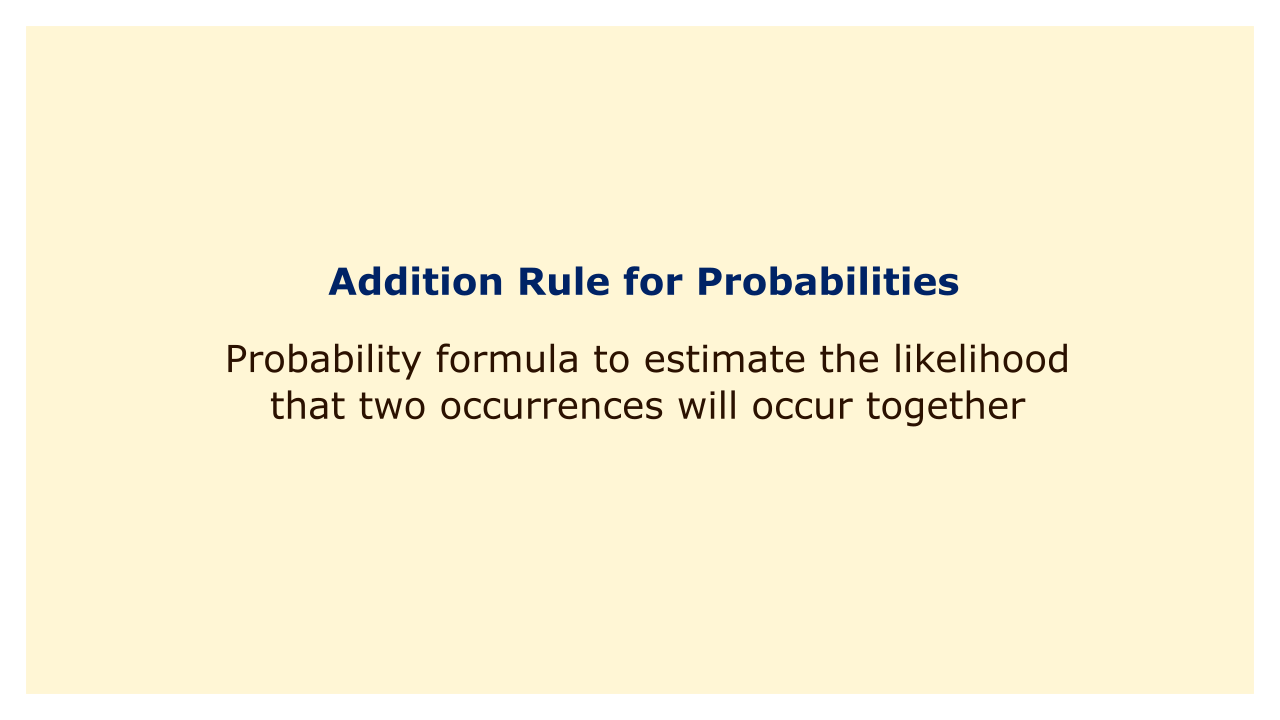Image: Moneybestpal.com

### The addition rule, which enables us to estimate the likelihood that two occurrences will occur together, is one of the most crucial ideas in probability theory.

The occurrence of either A or B, or both, is the event signified by the union of two occurrences A and B, A B. For instance, if event A is the flip of a coin and event B is the appearance of an even number on the die, then event A B is the occurrence of either the coin's landing on heads or the appearance of an even number on the die, or both.

According to the addition rule, the probability of A B is equal to the sum of A and B's probabilities, less the likelihood that they would intersect. The event where both A and B happen is shown by A B, which stands for the intersection of two events. For instance, if A and B are as described above, then A B would occur if both the coin and the dice produced an even number.

Mathematically, we can write the addition rule as:

P(A ∪ B) = P(A) + P(B) - P(A ∩ B)

Because it appears in both P(A) and P(P), we must subtract P(A B) to prevent counting it twice (B). The probability of receiving a head or an even number, for instance, when rolling a die and a coin together is 0.5 + 0.5 - 0.25, or 0.75, where 0.25 is the probability of getting both a head and an even number.

The addition rule can be extended to more than two events, by applying it repeatedly. For example, if we have three events A, B, and C, then we can write:

P(A ∪ B ∪ C) = P(A) + P(B) + P(C) - P(A ∩ B) - P(A ∩ C) - P(B ∩ C) + P(A ∩ B ∩ C)

The general formula for n events is:

P(A1 ∪ A2 ∪ ... ∪ An) = ΣP(Ai) - ΣP(Ai ∩ Aj) + ΣP(Ai ∩ Aj ∩ Ak) - ... + (-1)^n+1P(A1 ∩ A2 ∩ ... ∩ An)

where Σ means summation over all possible combinations of indices.

Many issues concerning event probability can be solved using the addition rule. For instance, let's say we want to determine the likelihood that a card picked from a typical 52-card deck would be either a spade or a face card (jack, queen, or king). We can use the addition rule as follows:

= 13/52 + 12/52 - 3/52

= 22/52

= 11/26

In this case, we have 13 spades, 12 face cards, and 3 spades that are also face cards in the deck.

The addition rule also has a special case when the events are mutually exclusive, meaning they cannot occur at the same time. In this case, their intersection is empty, so P(A ∩ B) = 0. Therefore, we can simplify the addition rule to:

P(A ∪ B) = P(A) + P(B)

For example, if we toss a coin twice, the events "first toss is heads" and "second toss is tails" are mutually exclusive, since they cannot happen together. So,

P(first toss is heads or second toss is tails) = P(first toss is heads) + P(second toss is tails)

= 0.5 + 0.5

= 1

In conclusion, the addition rule is an essential tool for determining the likelihood that two occurrences will occur together. According to this rule, the likelihood of two events coming together is equal to the sum of their individual probabilities less the probability of their intersection. By employing iterative application, it can be expanded to encompass more than two occurrences. In the case of the events being mutually exclusive, it also has a simplified version.
Tags